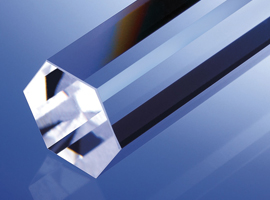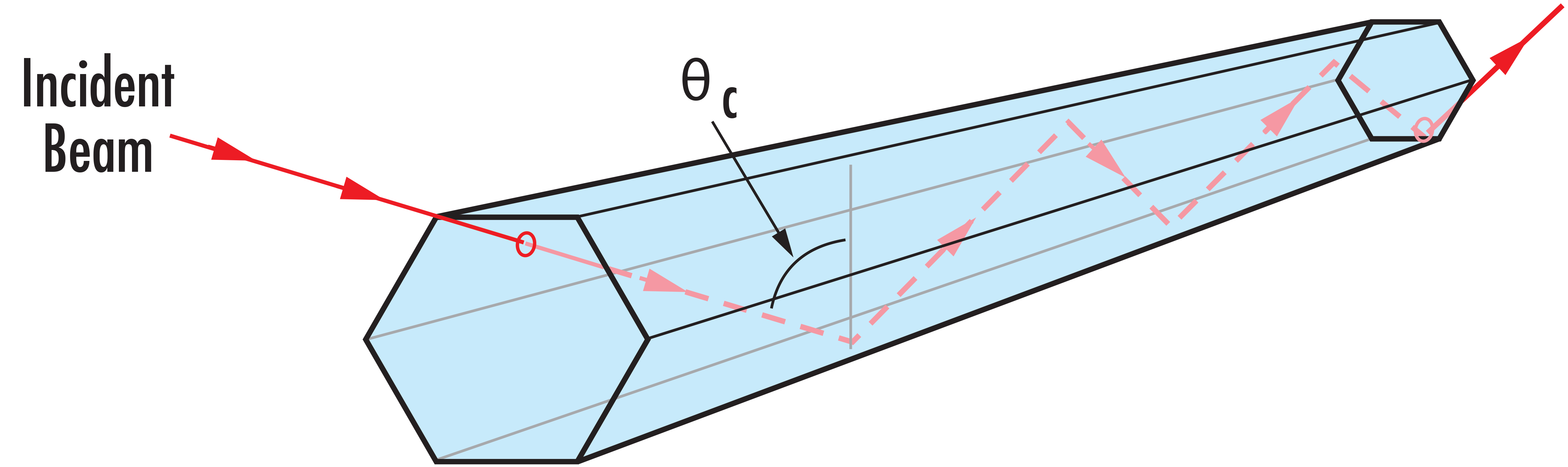# How to Select the Right Light Pipe Homogenizing RodMuch like optical fibers, light pipe homogenizing rods utilize total internal reflection (TIR) to transmit light from the entrance to the exit of the light pipe. The substrate’s refractive index is the only factor that influences the light pipe’s critical angle, which defines the angle of acceptance at which TIR will occur. Light pipes designed specifically for high NA, standard NA, and low NA light sources will have the same acceptance angle if designed with the same substrate.

The critical angle $\small{\left( \theta_c \right)}$ calculated using Equation 1:

(1)$$\theta_c= \sin^{-1} \! \left(\frac{1}{n}\right)$$

In the case of an N-BK7 light pipe, the index of refraction at Helium’s d-line of 587.9nm is 1.517, resulting in a $\small{ \theta_c }$ of 41°. If the incident angle is greater than $\small{\theta_c }$, TIR will occur and light will be transmitted throughout the light pipe (Figure 1).

Since light rays enter a light pipe at a variety of incident angles, the number of reflections inside the light pipe differs from ray to ray. The smallest incident angle, which is equal to $\small{ \theta_c }$, will have more reflections than light entering at an incident angle that is many times larger than $\small{ \theta_c}$ within the same length light pipe (Figure 2).

Due to low NA light sources having a larger number of rays greater than $\small{\theta_c}$ than high NA light sources, low NA light pipes are longer than high NA light pipes and are recommended for light sources with narrow beam divergence.

Note: Light pipes are not designed for use with collimated laser sources. To homogenize collimated light sources, Microlens Arrays or flat-top laser beam shapers are recommended. Light pipes are ideal for homogenizing polychromatic sources that emit non-collimated light.##### Figure 1: Total Internal Reflection (TIR) in a Light Pipe Homogenizing Rod